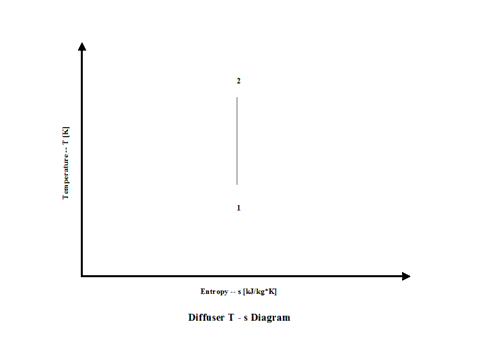top of page

### Diffuser Analysis

Diffuser

Introduction

This section provides an isentropic diffuser analysis when the working fluid is air.

Analysis

In the presented diffuser analysis, only air is considered as the working fluid behaving as a perfect gas -- specific heat has a constant value.  Ideal gas state equation is valid -- pv = RT.

Air enters a diffuser at point 1 and it exits the diffuser at point 2.  Working fluid inlet velocity gets reduced to zero resulting in the stagnation temperature and pressure inrease.  Isentropic process is considered with no entropy change.

Figure 1 contains a diffuser schematic layout.Figure 1 - Diffuser Schematic Layout

Figure 2 presents a diffuser temperature vs entropy diagram.Figure 2 - Diffuser Temperature vs Entropy Diagram

Figure 3 presents diffuser performance -- stagnation over static temperature and pressure ratio values as a function of the Mach Number.  Only subsonic diffuser operation is considered.  It should be noted that air enters the diffuser at the static conditions of 298 [K] and 1 [atm] of absolute pressure.Figure 3 - Diffuser Performance

One can notice that diffuser stagnation over static temperature and pressure ratio values increase with an increase in the Mach Number.

Assumptions

Working fluid is air. There is no friction and heat transfer. Isentropic process -- there is no entropy change.  Ideal gas state equation is valid -- pv = RT.  Air behaves as a perfect gas -- specific heat has a constant value.

Governing Equations

Tt/T = (1 + M^2(k - 1)/2)
p
t/p = (1 + M^2(k - 1)/2)^
ϰ/(ϰ-1)
T
t/T = (pt/p)^(
ϰ-1)/ϰ
T
t = T + v^2/(2cp)
v
s = (
ϰRT)^1/2
M = v/v
s

ϰ = cp/cv
cp - cv = R
pv = RT

Input Data

T1 = 298 [K]
p
1 = 1 [atm]
R = 0.2867 [kJ/kg*K]
c
p = 1.004 [kJ/kg*K]

ϰ = 1.4 [/]

M = 0.29, 0.58 and 0.95 [/]

Results

Diffuser Performance vs Inlet Mach Number
Diffuser Inlet Static Temperature = 298 [K] and Presuure = 1 [atm]

Inlet Mach
Number

[/]

0.29

0.58

0.95

Stagnation/Static
Temperature Ratio

[/]

1.017

1.067

1.182

Stagnation/Static
Pressure Ratio

[/]

1.06

1.25

1.80

Conclusions

Diffuser stagnation over static temperature and pressure ratio values increase with an increase in the Mach Number.

References

JANAF Thermochemical Data - Tables, 1970

bottom of page